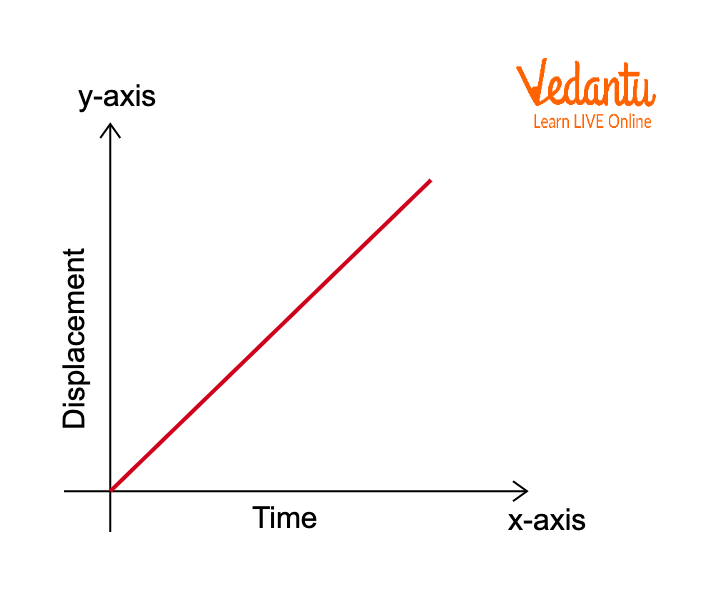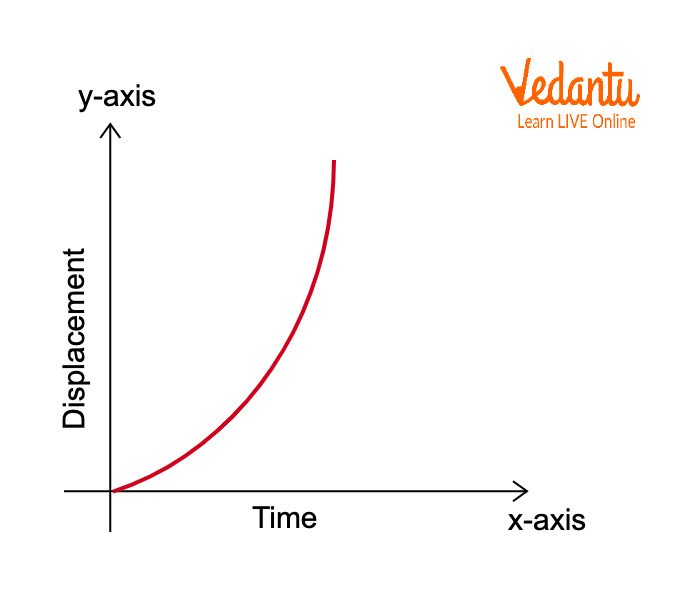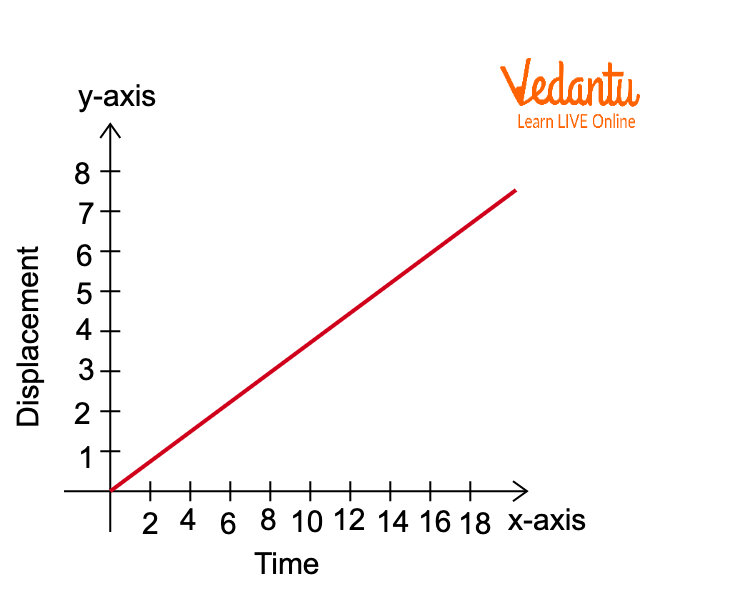Courses
Courses for Kids
Free study material
Free LIVE classes
More

# Displacement-Time Graph and Velocity-Time Graph for JEELIVE
Join Vedantu’s FREE Mastercalss

## What is Displacement?

Displacement of an object is defined as the change in position of the object. It is a vector quantity which means that it has a direction and a magnitude. Displacement can be written as the equation $\Delta x={{x}_{f}}-{{x}_{0}}$ where ${{x}_{f}}$ is the final position of the object and ${{x}_{0}}$ is the initial position of the object. The displacement-time graph tells us the distance covered by a moving object with respect to its direction at a certain time.

## Displacement-Time Graph

The displacement-time graph of a moving object tells the distance covered by a moving object with a change in time. The displacement is a vector quantity. The slope or the gradient of this graph indicates the object's velocity. The displacement-time graph is also known as the position-time graph, which represents the motion of an object. Here, in the graph, the moving object’s displacement is shown on the y-axis and is a dependent variable, whereas the time is shown on the x-axis and is an independent variable.

The correlation between the displacement and the velocity of the object gives rise to different types of slope shapes in the displacement-time graph. For example, if the displacement of the moving object increases with time, the graph shows a constant positive velocity. On the other hand, if the displacement of the moving object decreases with the duration of time, the graph shows a constant negative velocity.

## Displacement-Time Graph for Uniform Motion

A uniform motion is the type of motion in which the object moves in a straight line at a constant speed. The velocity of the moving object is constant throughout the motion.

Displacement-time graph of a uniform motion is the graph between the displacement and time when the motion of an object is uniform, which means the object's velocity is constant. This graph gives a straight line with a non-zero slope.Displacement-Time Graph for Uniform Motion

## Displacement-Time Graph for Uniform Acceleration

Acceleration is defined as the rate of change of velocity with respect to time. So, uniform acceleration of an object can be defined as the amount of change of velocity in equal intervals of time. The rate of change of velocity, in this case, is constant.

The displacement-time graph for uniform acceleration is a graph that gives a curved or a parabolic line because the rate of change of velocity in equal intervals of time changes as the moving object accelerates.Displacement-Time Graph for Uniform Acceleration

## Types of Displacement-Time Graphs

• ### When the Object is at Rest

An object is at rest, which means that the velocity of the object is zero, which indicates that the difference between the position of the object at different times will be zero because the object is not moving. Therefore, the slope of the graph will be zero. The graph will give us a straight line that is parallel to the x-axis of the graph, which is depicted by time.Displacement-Time Graph for Object at Rest

• ### When Velocity is Constant

When the velocity of the moving object is constant, the difference between the position of the objects at different times will not be zero and may give us a constant value, which means that the slope of the graph will not be zero, therefore, the displacement-time graph gives us a graph of uniform motion. This graph depicts a straight slanting line with a non-zero slope.Displacement-Time Graph for Constant Velocity

• ### When the Rate of Change of Velocity is Constant

We know that acceleration is defined as the rate of change of velocity, therefore, in this case, the acceleration of the moving object is constant. The graph indicates that the acceleration of a moving object is constant, and it gives the shape of a parabola.Displacement-Time Graph for Constant Rate of Change of Velocity

• There can be cases where the moving object is moving backward, thus, the slope of the displacement-time graph, in that case, will be negative.

• ## Displacement-Time Graph Examples

Here we take an example of uniform motion:

Rahul is driving a car with a constant speed that is indicated by the speedometer and he notices that the time taken by a car to travel one kilometer is 2 minutes.

He prepares a table that consists of the time taken in to travel every kilometer.

 Distance (in KM) 1 2 3 4 5 6 7 Time (in minutes) 2 4 6 8 10 12 14

Using the table, drawing the graph that shows the straight line and that the car travels with uniform velocity. Using the graph we can find the speed of the car by using the formula:

$\text{Speed}=\dfrac{\text{Final position}-\text{Initial position}}{\text{time}}$

For the given condition the speed of the car is:

Let us assume that the final position of the car is at 7 Km

And the initial position of the car is at 1 Km

The time taken by car is 12 minutes.

So, $\text{Speed}=\dfrac{\text{Final position}-\text{Initial position}}{\text{time}}$

$\text{speed}=\dfrac{7-1}{12}\times 60$

$\text{speed}=30km/h$Displacement vs Time Graph of Numerical

## Conclusion

Displacement-time graph establishes a relationship between the displacement covered by the moving object with respect to time. It tells us the distance covered by a moving object with a change in time. The displacement is a vector quantity. The slope or the gradient of this graph gives the velocity of the object. There are many cases for which a displacement-time graph can be drawn, such as the displacement-time graph for uniform motion, constant velocity, and uniform acceleration. This gives us different outcomes of the graphs.

Last updated date: 26th Sep 2023
Total views: 2.4k
Views today: 0.02k

## FAQs on Displacement-Time Graph and Velocity-Time Graph for JEE

1. What does the slope of the displacement-time graph indicate?

The slope or gradient of the displacement-time graph indicates the velocity of the moving object. The velocity of an object is defined as the rate of change of displacement. As displacement is a vector quantity, thus velocity is also a vector quantity which further means that it also has a direction as well as magnitude. The change in position of an object at a certain time is called velocity. Therefore, the slope of the displacement-time graph is velocity.

2. When the slope of a displacement-time graph is positive, what does it indicate?

If the slope of a moving object in a displacement-time graph is positive, it indicates that the moving object is moving away from the starting point, which means the displacement of the moving object is increasing with respect to time. The increase in the displacement of the object with time increases, it shows a constant positive velocity. Furthermore, if the slope is negative, it means that either the displacement of the object with time is decreasing, or the object is reverting back to its initial point.

3. What is the shape of the displacement-time graph for a uniform motion?

A uniform motion is defined as the type of motion in which an object moves in a straight line at a constant speed. If the speed or the velocity of the moving object is constant, it means the difference between the position of the moving object at different times will not be zero and will always give a constant value. Therefore, for a uniform motion, the displacement-time graph gives us a straight line with a non-zero slope, which means, the velocity of the object is not zero.